#### Gamma distribution calculator

The gamma distribution is a continuous probability distribution used to model a variety of real-life situations, such as the time between events in a Poisson process. In MS Excel, the Gamma distribution can be easily calculated by using the GAMMA.DIST function.

## Function Details

=GAMMA.DIST (x, alpha, beta, cumulative)

## Arguments of Function

• X (Required) – The value at which the gamma function would be evaluated
• Alpha / α (Required) – A parameter of the distribution used for determining the shape.
• beta / β (Required) – A parameter of the distribution for determining the rate

α and β are both must be greater than 1.

When α = 1, it corresponds to an exponential distribution.

When β = 1, it corresponds to the standard gamma distribution.

Cumulative (Required) – A logical value that determines the form of the function.

Cumulative =TRUE, it returns the cumulative distribution function

Cumulative =FALSE, it returns the probability density function.

## Stepwise Execution

Go to an empty cell and type =GAMMA.DIST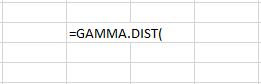1. Type in the value where we need to find the probability. This gives us the value of x. In the example below, x= 5
2. Type the alpha and beta value as the next parameters, both comma separated. Here, α = 4 & β = 3
3. Type True for cumulative distribution. Type False for probability density function.

## Formula & Result

=GAMMA.DIST(5, 4, 3, TRUE)

Result: 0.088267

=GAMMA.DIST(5, 4, 3, False)

Result: 0.048579

Graph Generation Cumulative Distribution Function (CDF) and Probability Density Function (PDF)

Take time T = 0 – n. In the example, time is taken from 0 to 20. Here value of T = value of x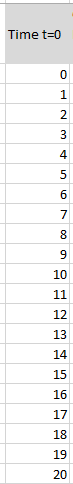Fix values for α = 4 & β = 3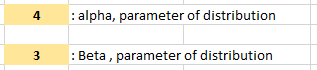## Cumulative Distribution function (CDF)

Formula: =GAMMA.DIST(D16, 4, 3, TRUE)

Probability Density function (PDF)

Formula: =GAMMA.DIST(D16, 4, 3, FALSE)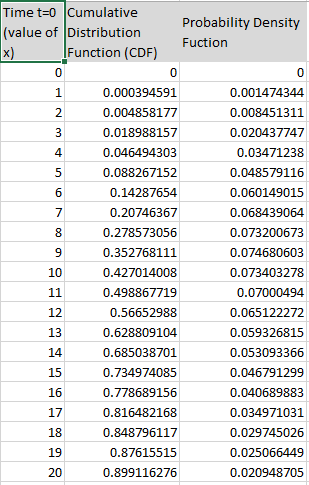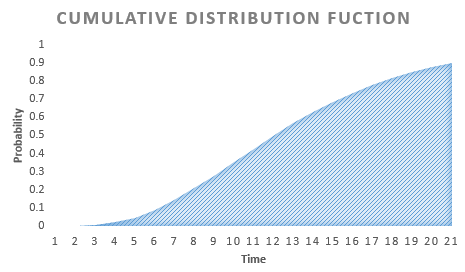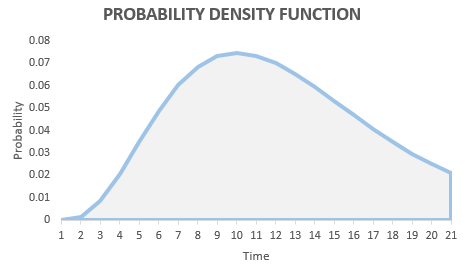### Errors

• #NUM! – Occurs under the following scenarios:

Value of x < 0

Value of α ≤ 0 or β ≤ 0

• #VALUE! – Occurs under following scenarios:

Any of the arguments – x, alpha, beta are non-numeric in nature.

The fourth parameter is not TRUE OR FALSE, unrecognized.

Note that the GAMMADIST function requires that the alpha parameter be greater than 0 and the beta parameter be greater than 0. If the parameters are not valid, the function will return the #NUM! error.

There are also other functions in Excel that can be used to model the gamma distribution, such as the GAMMAINV function, which calculates the inverse of the gamma cumulative distribution function, and the GAMMA function, which calculates the gamma function.

It’s important to carefully review the distribution of your data and choose the appropriate distribution function to model it accurately.